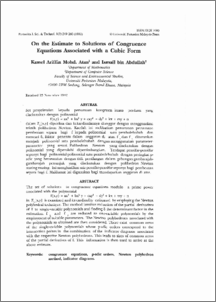# On the Estimate to Solutions of Congruence Equations Associated with a Cubic Form

## Citation

Mohd Atan, Kamel Ariffin and Abdullah, Ismail (1993) On the Estimate to Solutions of Congruence Equations Associated with a Cubic Form. Pertanika Journal of Science & Technology, 1 (2). pp. 249-260. ISSN 0128-7680

## Abstract

The set of solutions to congruence equations modulo a prime power associated with the polynomial f(x,y) = ax3 + bx2 y + cxy2 + dt + kx + my + n in Z [x,y] is examined and its cardinality estimated by employing the Newton polyhedral technique. The method involves reduction of the partial derivatives of f to single-variable polynomials and finding 8 the determinant factor in the estimation. f x and f yare reduced to one-variable polynomials by the employment of suitable parameters. The Newton polyhedrons associated with the polynomials so obtained are then considered. There exist common zeros of the single-variable polynomials whose p-adic orders correspond to the intersection points in the combination of the indicator diagrams associated with the respective Newton polyhedrons. This leads to sizes of common zeros of the partial derivatives of f. This information is then used to arrive at the above estimate.Preview
PDF
On_the_Estimate_to_Solutions_of_Congruence.pdfView Item